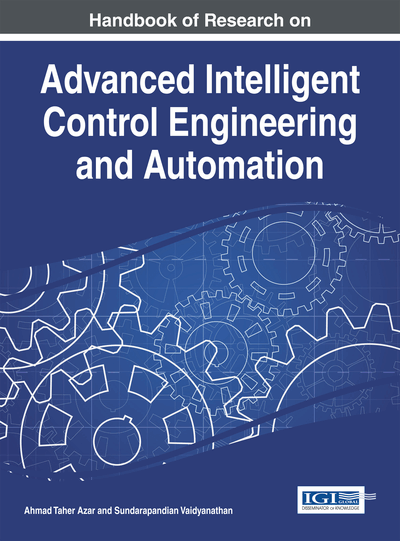# Fuzzy Adaptive Controller for Uncertain Multivariable Nonlinear Systems with Both Sector Nonlinearities and Dead-Zones

Abdesselem Boulkroune (University of Jijel, Algeria)
DOI: 10.4018/978-1-4666-7248-2.ch012
Available
\$33.75
List Price: \$37.50
10% Discount:-\$3.75
TOTAL SAVINGS: \$3.75

## Abstract

This chapter presents two fuzzy adaptive variable structure controllers for a class of uncertain multi-input multi-output nonlinear systems with actuator nonlinearities (i.e. with sector nonlinearities and dead-zones). The design of the first controller concerns systems with symmetric and positive definite control-gain matrix, while the design of the second one is extended to the case of non-symmetric control-gain matrix thanks to an appropriate matrix decomposition, namely the product of a symmetric positive-definite matrix, a diagonal matrix with diagonal entries +1 or -1, and a unity upper triangular matrix. An appropriate adaptive fuzzy-logic system is used to reasonably approximate the uncertain functions. A Lyapunov approach is adopted to derive the parameter adaptation laws and prove the stability of the closed-loop control system. Finally, some simulation results are carried out to show the effectiveness of the proposed controllers.
Chapter Preview
Top

## Introduction

Problems of process control are more and more complex as the involved systems are multivariable in nature and exhibit uncertain nonlinear behaviours. This explains the fact that only few engineering solutions are available. Thanks to the universal approximation theorem (Wang, 1994), some adaptive fuzzy control systems have been developed for a class of multivariable nonlinear uncertain systems (Ordonez & Passino, 1999; Chang, 2000; Tong et al., 2000; Chekireb et al., 2003; Golea et al., 2003; Li & Tong, 2003; Tong & Li, 2003; Labiod et al., 2005; Tong et al., 2005; Essounbouli et al., 2006). The stability of the underlying control systems has been investigated using a Lyapunov approach. The robustness issues with respect to the fuzzy approximation error and external disturbances have been enhanced by appropriately modifying the available adaptive fuzzy controllers. The corner stone of such a modification consists in a robust control term which is conceived using a sliding mode control design (Ordonez & Passino, 1999; Chekireb et al., 2003; Golea et al., 2003; Tong & Li, 2003; Labiod et al., 2005; Tong et al., 2005) or anbased robust control design (Chang, 2000; Tong et al., 2000; Li & Tong, 2003; Essounbouli et al., 2006). A key assumption in the available fuzzy adaptive control systems (Ordonez & Passino, 1999; Chang, 2000; Tong et al., 2000; Chekireb et al., 2003; Golea et al., 2003; Li & Tong, 2003; Tong & Li, 2003; Labiod et al., 2005; Tong et al., 2005; Essounbouli et al., 2006) is that the actuator dynamics may be reasonably approximated by a linear model. This is more an exception than a rule in the engineering practice.

The control problem of uncertain multi-input multi-output (MIMO) systems with input nonlinearities has received a remarkable attention because of those ubiquitous actuator nonlinearities, namely saturation, quantization, backlash, dead-zone and so on (Gutierrez & Ro, 1998; Hsu et al., 2004). It is well known that the existence of input nonlinearities may leads to poor performance or even instability of the control system. It is thereby more advisable to take into account the actuator nonlinearities in the control design as well as the stability analysis. Decentralized variable-structure controllers have been proposed in (Hsu, 1999; Shyu et al., 2003; Shyu et al., 2005) for a class of systems with input sector nonlinearities and/or dead-zones. In (Hsu et al., 2004; Niu & Ho, 2006), the authors have designed sliding mode control systems for nonlinear MIMO systems subject to both sector nonlinearities and dead-zones. The underlying results suffer from two fundamental limitations. Firstly, the considered class of systems is relatively reduced. Secondly, the gain reduction tolerances of the nonlinear dead-zones and upper bounds of uncertain nonlinear functions are required to be known.

## Key Terms in this Chapter

Fuzzy Control: A means of expressing an operator’s knowledge of controlling a process with a set of fuzzy IF-THEN rules and linguistic variables.

Multi-Input–Multi-Output System (MIMO): Also known as multivariable system. A system that can transform two or more input signals to two or more output signals.

Actuator Nonlinearities: They are due to some physical limitations and include friction, dead zone, backlash, hysteresis and saturation.

Fuzzy Universal Approximation Theorem: Any sufficiently smooth function can be approximated arbitrarily closely on a compact set using a fuzzy system with appropriate structure.

Matrix (SDU) Decomposition: A matrix factorization into a product of a symmetric positive definite matrix, a diagonal matrix whose elements are +1 or -1 and a unity upper triangular matrix.

Variable Structure System: It consists of a set of continuous subsystems together with a suitable switching logic.

Lyapunov’s (Second) Approach: The method of stability assessment that relies on the use of energy-like functions without resorting to direct solution of the associated evolution equations.

Adaptive Control: The control parameters in this approach are continuously and automatically adjusted in response to be measured process variables to achieve near-optimum system performance.

## Complete Chapter List

Search this Book:
Reset